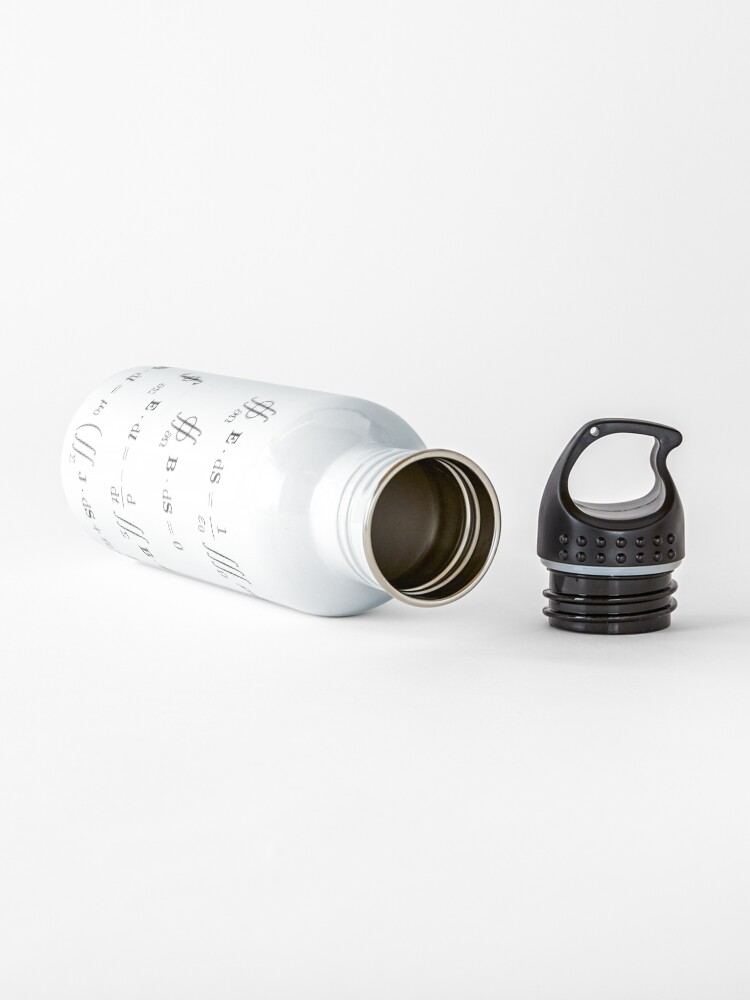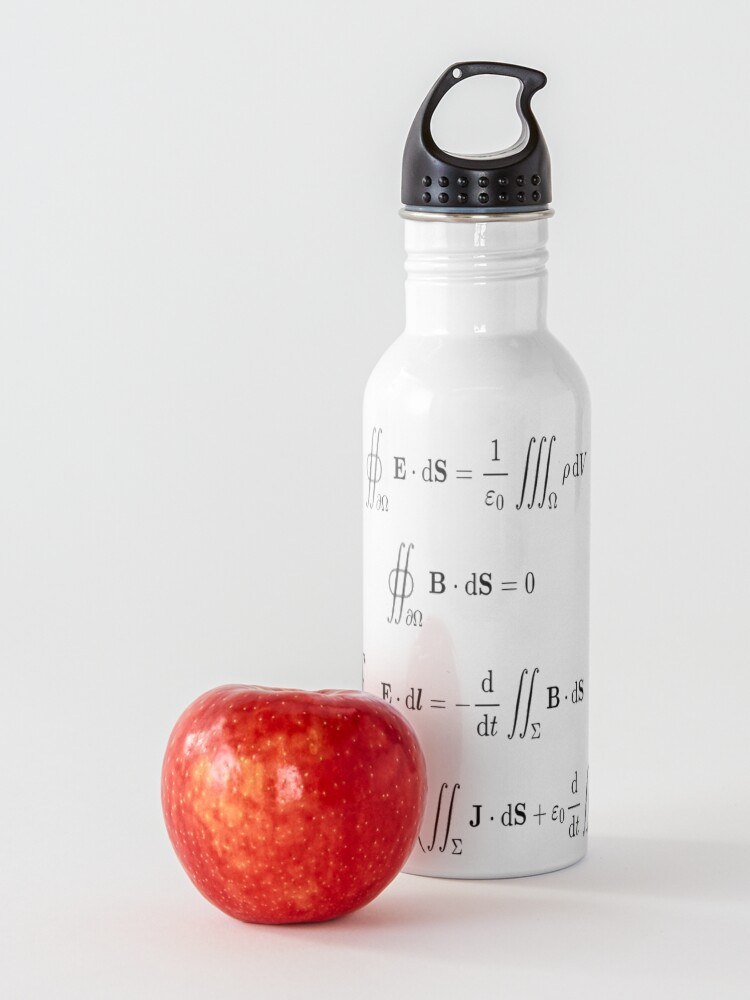Features

• Stainless steel bottle holds 20 oz (590ml) and comes with a leak proof cap
• Lightweight, durable and easy to carry
• Reusable, so it's safe for the planet
• 360 degree full-wrap vibrant print

ReviewsMaxwell's equations, #Maxwells, #equations, #MaxwellsEquations, Maxwell, equation, MaxwellEquations, #Physics, Electricity, Electrodynamics, Electromagnetism
Designed and sold by znamenski
Maxwell's equations are a set of partial differential equations that, together with the Lorentz force law, form the foundation of classical electromagnetism, classical optics, and electric circuits. The equations provide a mathematical model for electric,

Maxwell's equations, #Maxwells, #equations, #MaxwellsEquations, Maxwell, equation, MaxwellEquations, #Physics, Electricity, Electrodynamics, Electromagnetism Water Bottle

Designed and sold by znamenski

\$18.88
Delivery
Express by January 26
Standard between January 26